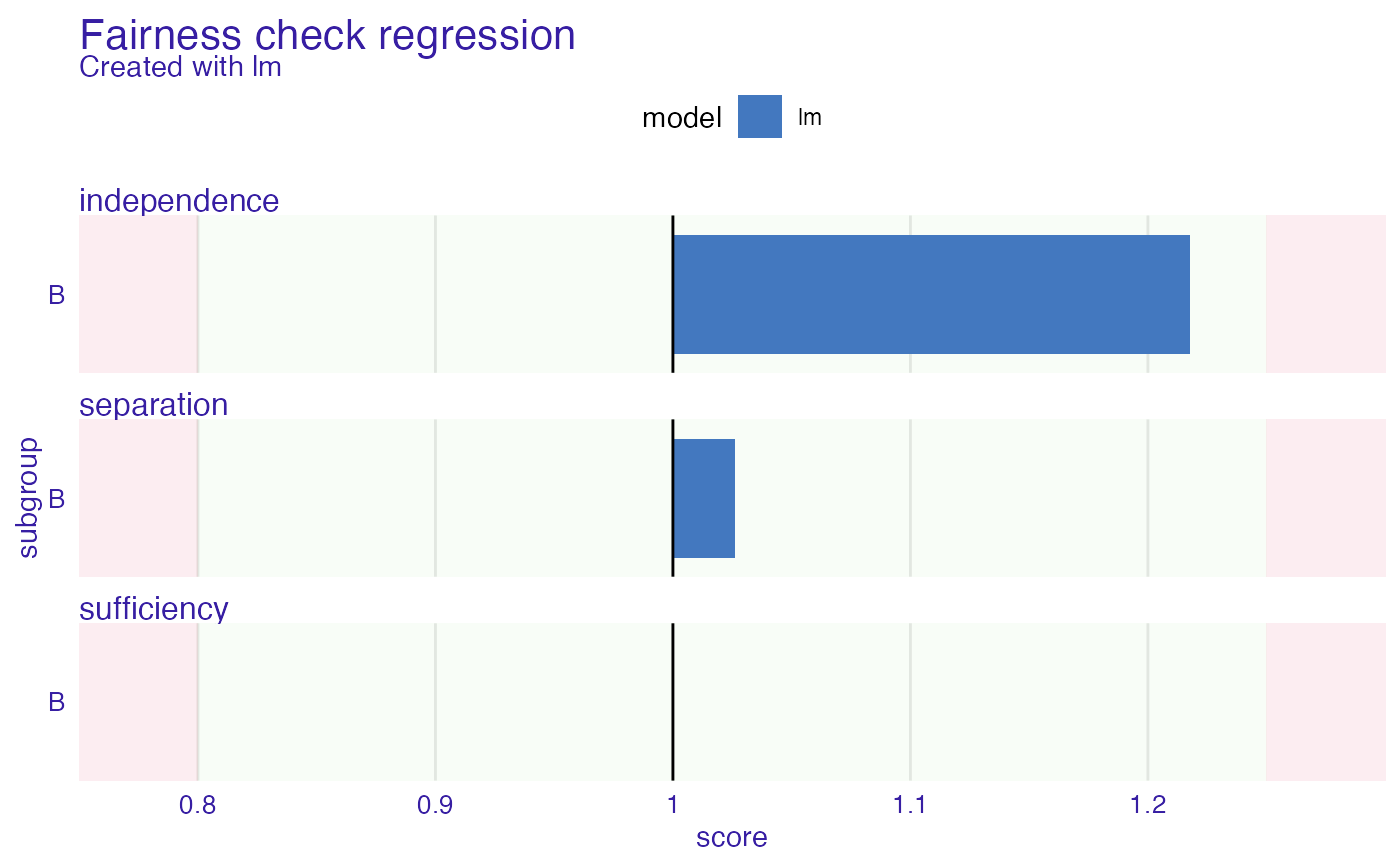Please note that this is experimental approach. Plot fairness check regression enables to look how big differences are between base subgroup (privileged) and unprivileged ones. If bar plot reaches red zone it means that for this subgroup fairness goal is not satisfied. Multiple subgroups and models can be plotted. Red and green zone boundary can be moved through epsilon parameter, that needs to be passed through fairness_check.

# S3 method for fairness_regression_object
plot(x, ...)

Arguments

x fairness_regression_object object other plot parameters

ggplot2 object

Examples

set.seed(123)
data <- data.frame(
x = c(rnorm(500, 500, 100), rnorm(500, 400, 200)),
pop = c(rep("A", 500), rep("B", 500))
)

data$y <- rnorm(length(data$x), 1.5 * data$x, 100) # create model model <- lm(y ~ ., data = data) # create explainer exp <- DALEX::explain(model, data = data, y = data$y)
#> Preparation of a new explainer is initiated
#>   -> model label       :  lm  (  default  )
#>   -> data              :  1000  rows  3  cols
#>   -> target variable   :  1000  values
#>   -> predict function  :  yhat.lm  will be used (  default  )
#>   -> predicted values  :  No value for predict function target column. (  default  )
#>   -> model_info        :  package stats , ver. 4.1.1 , task regression (  default  )
#>   -> predicted values  :  numerical, min =  -269.546 , mean =  681.4906 , max =  1444.562
#>   -> residual function :  difference between y and yhat (  default  )
#>   -> residuals         :  numerical, min =  -302.6659 , mean =  -9.167582e-14 , max =  332.7938
#>   A new explainer has been created!

# create fobject
fobject <- fairness_check_regression(exp, protected = data$pop, privileged = "A") #> Creating fairness regression object #> -> Privileged subgroup : character ( Ok ) #> -> Protected variable : factor ( changed from character ) #> -> Fairness objects : 0 objects #> -> Checking explainers : 1 in total ( compatible ) #> -> Metric calculation : 3/3 metrics calculated for all models #> Fairness regression object created succesfully #> # results fobject #> #> Fairness check regression for models: lm #> #> lm passes 3/3 metrics #> Total loss: 0.243362 #> plot(fobject)# \donttest{ model_ranger <- ranger::ranger(y ~ ., data = data, seed = 123) exp2 <- DALEX::explain(model_ranger, data = data, y = data$y)
#> Preparation of a new explainer is initiated
#>   -> model label       :  ranger  (  default  )
#>   -> data              :  1000  rows  3  cols
#>   -> target variable   :  1000  values
#>   -> predict function  :  yhat.ranger  will be used (  default  )
#>   -> predicted values  :  No value for predict function target column. (  default  )
#>   -> model_info        :  package ranger , ver. 0.13.1 , task regression (  default  )
#>   -> predicted values  :  numerical, min =  210.6774 , mean =  681.3779 , max =  987.8878
#>   -> residual function :  difference between y and yhat (  default  )
#>   -> residuals         :  numerical, min =  -668.0995 , mean =  0.1126996 , max =  629.5469
#>   A new explainer has been created!

fobject <- fairness_check_regression(exp2, fobject)
#> Creating fairness regression object
#> -> Privileged subgroup		: character ( from first fairness object  )
#> -> Protected variable		: factor ( from first fairness object  )
#> -> Fairness objects		: 1 object (  compatible  )
#> -> Checking explainers		: 2 in total (  compatible  )
#> -> Metric calculation		: 3/3 metrics calculated for all models
#>  Fairness regression object created succesfully
#>

# results
fobject
#>
#> Fairness check regression for models: ranger, lm
#>
#> ranger passes 0/3 metrics
#> Total loss:  2.086092
#>
#> lm passes 3/3 metrics
#> Total loss:  0.243362
#>

plot(fobject)# }